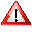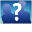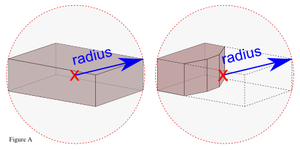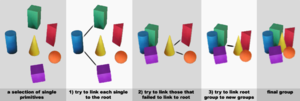This article is out of date! This formula was used with Havok 4 but was replaced by a much simpler one. See Linkability Rules for current information.Trivia: If you are curious about how linking worked with Havok 1, see this archived forum post.

Determining whether or not a set of prims/objects is linkable is somewhat complex. Generally, larger prims and objects may have greater distances between them to become linked, with a minimum of 2 meters and a maximum of 54. The distance depends on the position and the X/Y/Z size of each prim, but not their shapes, rotations, size changes due to cuts, hollows, etc.

## In Brief

• If the distance between two or more objects is too large, they cannot be linked.
• The allowed distance is larger for large objects, and smaller for small objects.
• The real rules are quite complicated.

## Quick overview

Kelly's Quick Overview:

• Two pieces can link if their bounding boxes would completely fit within a "Linkability Sphere".
• The size of the Linkability Sphere is between 2m and 54m diameter, depending on the scale of the two pieces being linked.
• Specifically the size is 3 times the sum of both object's bounding sphere radius plus 2 meters, up to a maximum of 54m.
• If linking more than two pieces, treat any 2 pieces that can link by the above rule as 1 piece whose scale encompasses both pieces.
• Repeat until everything is linked.

At the small extreme: two tiny prims can be linked if they are within about 2 meters from each other.

At the large extreme: the largest linked object that can be created must fit within a sphere with a diameter of 54 meters.Figure A: The bounding spheres of two example prims, one of which has been cut. The two spheres are identical in size.
• Whether two prims can be linked depends only on each prim's X/Y/Z scale and position. Other properties (such as rotation, hollow, cut, sculpt, etc.) are ignored. This means that linking calculations are done as if every prim were a rectangular box that is stretched but otherwise undistorted (i.e. with the X, Y, and Z sizes the same as the original prim, but everything else 'normal').
• Editing and selecting any object and selecting the 'stretch' tool will display this "local bounding box" for that object.
• Exception for megaprims: If any side is more than 10 meters, treat that side as if it were 10 meters.

A way to visualize the procedure:

1. Imagine transforming every prim in every object into the aforementioned boxes.
2. For each existing (linked-together) object, imagine the smallest sphere that totally encloses the bounding boxes of the prims which belong to it. (For individual prims, just enclose that one box.)
3. Add another meter to each sphere's diameter, then double that diameter. (Don't move the centers of the spheres.)
4. You can link objects if their spheres touch or intersect. Link everything that can be linked at this point.
• Exception: If any new object would need a sphere of bigger than 54 meters to enclose all its boxes, you can't make that object.
5. Repeat this procedure with the newly linked objects until you can't link any more.

## The details

Consider two primitives, A and B. Whether they can be linked or not is determined by measuring the span from the edge of one object's bounding sphere to the far opposite edge of the other's bounding sphere and comparing that value to the maximum linkability span which is a function of the radii of the two bounding spheres:

```   (1)     max_link_span = minimum( 3 * (radius_A + radius_B) + LINK_BONUS, LARGEST_MAX )
```

where:

```   (3)     LINK_BONUS = 2.0 meters
(4)     LARGEST_MAX = 54.0 meters
(5)     radius_X = radius of the primitive X's bounding sphere (Figure A)
(6)     minimum(C, D) = C if less than D, otherwise D
```

If the measured span of the two bounding spheres is less than or equal to max_link_span then the corresponding primitives can be linked. Put in mathematical terms:

```   (7)     A_can_link_to_B = ( length(center_A - center_B) + radius_A + radius_B <= max_link_span )
```

The bounding sphere is the smallest sphere that totally encloses the primitive's local bounding box.

The local bounding box is centered at the primitive's geometric center and has sides that are equal to the primitive's scale. One exception to this rule is that megaprim scale components greater than 10 meters are clamped to 10.

The geometric center of the primitive is its local symmetric center prior to any cut, shear, twist, taper, or hollow operations.

Note that a primitive's bounding sphere is not necessarily the tightest sphere possible for its shape, unless it is a simple box. The bounding sphere depends only on the primitive's position and scale, so any severly cut and hollowed primitive will be significantly smaller than its bounding sphere, and not necessarily near the center. Also, a primitive with twist and/or shear may have corners that extend outside of its bounding sphere. Since the linkability rules depend only on the bounding sphere, which is ultimately dependent only on the primitive's position and scale, the linkability of two prims is independent of changes to form and rotation.

TODO -- we need new figures _B and _C for two linkable prims and two linkable multi-prim objects.Figure B: Example of how groups of primitives are linked to a root primitive for three or more objects.

The rules governing the linkability of multi-prim objects is very similar to the two-primitive case. The same formulae (1) and (7) apply, but the bounding spheres of multi-prim objects are the smallest spheres that completely contain all of the bounding spheres of the corresponding primitives.

When linking three or more objects the algorithm iterates over the candidate objects until all linkable pieces have been found. First the root object is tested against each candidate object and the larger bounding sphere is recomputed after a successful link. Then any unlinked pieces are tested between themselves and merged into larger collections according to the formulae. The root object is then re-tested against the modified candidates and the process continues until all objects are linked, or no new links have been found.

### Failure modes

If an unlinkable set is tested by the linkability algorithm then the final subset of linkable parts is determined by the order in which the candidates were submitted. The trivial proof for this is to consider a root primitive in the middle of an infinite grid of other primitives. It can't link to everything, but it were first tested against all primitives west of it the final linkable subset of that first operation might not link to any primitives to the east because of the LARGEST_MAX requirement (4). If the primitives to the east were tested first then the final result would be different.

If a linkable set is tested by the linkability algorithm then the final subset of linkable parts is NOT affected by the order in which the candidates were submitted. That is, if just the linkable subsets of the failure modes above are tested for all permutations of sequence they will always link. The proof of this is left as an exercise for the reader.

### Formulas

Radius of the Bounding Sphere: SQRT(x^2 + y^2 + z^2)/2

Distance between the centers (two prims): Minimum of ((3 * (radius_A + radius_B) + LINK_BONUS) - (radius_A + radius_B)) and 54 - (radius_A + radius_B). If the 54 meters maximum is not reached the distance between centers formula can be condensed to:

SQRT(Xa^2 + Ya^2 + Za^2) + SQRT(Xb^2 + Yb^2 + Zb^2) + LINK_BONUS

### Examples

#### 2 very small prims

LARGEST_MAX = 54.0 meters

2.06 is less than 54

#### one large prim and one small prim

radius_A = 8.66m (This is a 10x10x10m prim of any shape type)

LARGEST_MAX = 54.0 meters

28.01 is smaller than 54.0

Note this is NOT the maximum distance between the two centers. This is the maximum distance between the farthest edges of A and B.

#### 2 very large prims

The diameter of a bounding sphere is the square root of x^2 + y^2 + z^2 thus, the diameter of a 10m x 10m x 10m prim is the square root of (100+100+100) = ~17.3m and the diameter of a 10m x 1m x 1m prim is square root (100+1+1) = ~10.1m (The type of prim doesn't matter for this calculation. We only care about the dimensions.) Let's take the case of two 10m x 10m x 10m prims.

LARGEST_MAX = 54.0 meters

53.96 is smaller than 54

Note this is NOT the maximum distance between the two centers. The center of each prim is actually 8.66m meters inside the bounding sphere, so the max distance between the centers is approximately 36.64m.

Distance between centers: SQRT(Xa^2 + Ya^2 + Za^2) + SQRT(Xb^2 + Yb^2 + Zb^2) + LINK_BONUS

SQRT(10^2+10^2+10^2) + SQRT(10^2+10^2+10^2) + 2

SQRT(300) + SQRT(300) + 2

17.32 + 17.32 + 2 = 36.64m

## Bugs

### Some stuff that could be linked in Havok1 cannot be linked in Havok4

The Havok4 linkability limits are larger that Havok1 for most prim collections, however there is indeed a set of objects that were linkable in Havok1 that are NOT linkable in Havok4.

Havok1 used an "axis aligned bounding box" (AABB) algorithm, and it turns out that the span (distance from one corner of the AABB to the opposite corner) of the maximum box in Havok1 is longer than the diameter of the maximum bounding sphere in Havok4.

The maximum AABB was actually a box with sides of about 32 meters ==> span was 55.4 meters. So the Havok1 box almost fits inside the 54 diameter sphere used in Havok4... but has some corners that stick out very slightly ==> any house that just barely fits inside the AABB might not link in Havok4.

Note that "axis aligned" means that if you were to rotate your house... the maximum box would NOT rotate since it was always aligned with the axes of the world (East, North, and Up), and your house would not fit inside the box in its rotated state, and would unlink later after the region suffered a shutdown/load cycle (this was a bug in Havok1), or if you were to take it to inventory in its rotated state and then rez that inventory (it would show up unlinked).

### When I link two prims the maximum distance between them does NOT agree with the formula above.

Make sure you are measuring the span of the final bounding sphere and not the distances between the centers of the two primitives.